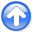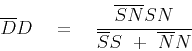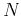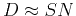Spitz makes a better assumption for the signal PEFNext: Signal and noise separation Up: Claerbout & Fomel: Estimating Previous: INTRODUCTION

# BASIC THEORY

Signal spectrum plus the noise spectrum gives the data spectrum. Since a prediction-error filter tends to the inverse of a spectrum we have(1)(2)

or(3)

Now we are ready for the Spitz approximation. Spitz builds his applications upon the assumption that we can estimateandfrom suitable chunks of raw data. His result may be obtained from (3) by ignoring its denominator gettingor(4)

Ignoring the denominator in equation (3), is not so terrible an approximation as it might seem. Remember that PEFs are important where they are small because they are used as weighting functions. Where weighting functions are small, solutions are expected to be large. Although Claerbout's assumptionmight be somewhat valid for signal and data spectra, it is much less valid for their PEFs. In practice, signal unpolluted with noise is usually not available. Even a very good chunk of data tends to yield a poor estimate of the signal PEFbecause the holes in the signal spectrum are easily intruded with noise.

Obviously the major difference betweenandis where the noise is large. Thus it is for organized and predictable'' noises (small) where we expect to see the main difference.

Theoretically, we need not make the Spitz approximation. We could solve (1) forby spectral factorization. Although theobtained would be more theoretically satisfying, there would be some practical disadvantages. Getting the signal spectrum by subtracting that of the noise from that of the data leaves the danger of a negative result (which explodes the factorization). Thus, maintaining spectral positivity would require extra care. All these extra burdens are avoided by making the Spitz approximation. All the more so in applications with continuously varying estimates.Spitz makes a better assumption for the signal PEFNext: Signal and noise separation Up: Claerbout & Fomel: Estimating Previous: INTRODUCTION

2013-03-03